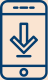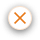#### Helping you invest with confidence# Basic Math You Should Know Before Investing in Stocks

21 Dec 2022 0 COMMENTPeople People often consider investing in the stock market a difficult task due to the involvement of numbers or advanced mathematics. Investing is one of the best ways to generate wealth over a long period of time, but many people find it difficult to formulate trading strategies, fundamental analysis, technical analysis and investing strategies. Although it is true that some investors make use of advanced mathematical models, many investors have achieved great success in the stock market using just basic math. Here is the basic stock market math that one should know before investing in stocks.

An investor looking to learn more about math for stock market should focus on the 3 main concepts:

• Basic arithmetic and algebra
• Compounding
• Probabilities

## Basic arithmetic

As an investor, it is necessary to be proficient in basic arithmetic like adding, subtracting, division and multiplication. Learning basic arithmetic can help in making use of certain algebraic equations, allowing you to invest smartly in the stock market.

Some of the most important stock market math formulas are:

1.  Future Value

As the name suggests, the future value equation can help you ascertain the future value of an investment after a period of time and the amount of money one needs to invest to achieve their financial goals.

The formula to find the future value of an asset is:

F = P * (1+R)t

where,

F: Future value

P: The present value of the investment that has been made by the investor

t: The number of periods for which the returns received will compound

R: The interest rate or rate of return

2. Return on Equity (ROE)

Another important equation in stock market math is to calculate the return on equity. By calculating the return on equity, it is easier for an investor to assess if the returns that are provided by the company are worth the investments that have been made. Although this can’t be the only factor to select a stock, it can be used to compare the ROE of other companies in the same industry.

ROE = (Net income/shareholder equity)

An investor can find the data of the income and shareholders’ equity in the company’s financial reports like the balance sheet and the profit and loss statement.

3. Total Return

The total return formula is used calculate to know the returns that an investment has generated. This formula also considers the dividend received in order to assess the total returns.

Total returns = {(Value of investment at the end of the year – value of investment at the start of the year) + dividends] / Value of investment at the beginning of the year

4.  P/E Ratio

One of the most essential ratios that is used by many while making investment decisions is the Price to Earnings Ratio or P/E ratio. This ratio helps understand if a company’s shares are overvalued or undervalued. Investors use this ratio to compare it with the industry average and its peers.

In order to calculate this ratio, the market price of the share is divided by the earnings per share. The earnings per share refer to the annual per-share earnings of the company.

P/E Ratio = Market price of share/earnings per share

## Compounding

While learning math for stock market, another important concept that needs to be understood is compounding.

In the world of investing, compounding refers to the process in which the returns that are generated by the investment are reinvested. These returns stay invested and start earning returns as well. Let’s look at an example to learn more about this.

Let’s assume an investment of Rs 5,000 earns a return of Rs 250 per year.

If these returns are not reinvested then at the end of 10 years, the total return will be Rs 2,500 and which will bring the value of the investment to Rs 7,500.

In the case of these returns being reinvested, the total return at the end of 10 years will be Rs 3,235 and the final value of the investment will be Rs 8,235.

Due to compounding, the returns were reinvested which earned returns as well. This led to a 9.3% increase compared to the returns not being reinvested. Compounding is especially helpful in the long term.

## Probabilities

Probabilities are a very helpful concept in math for stock market. Probabilities can help an investor can get an idea of what the odds are of an investment performing well. While making investment decisions, an investor has to consider various factors like the company’s management, business models, financial ratios etc. After assessing these factors, an investor can come up with a probability of an investment being successful. Depending on this probability, the investor will make an investment decision.

When investing, there is no certainty or guarantee that an investment will perform well therefore, probabilities can help an investor put their hard-earned money into stocks that have a higher probability of performing well. Moreover, understanding probability can also help you in risk management.

In conclusion, the math behind stock market is not complicated. Investors can make use of these concepts in order to make informed investment decisions and plan their finances accordingly.